# Difference between revisions of "Brownian motion"

Gravitation as binding force between Molecules to explain Brownian Motion in Multi Molecule Theory using Dynamic Universe Model

Nanobiotechnology is a wonderful multidisciplinary budding science have its roots in four main branches of science viz, Particle Physics, Nano Biology, and Micro chemistry and Engineering. This is Nano particle Physics portion which is forming the basis of the Nanobiotechnology. Until today the behaviour of fluid particles in Brownian motion are explained using Single molecule theory. But many questions remain for the last 400 years or so, how this Brownian motion happens? Why collisions happen between the Molecules? How the momentum is generated in the starting place? What are the are the trajectories of individual particles or molecules? The Physics and the calculations behind the force and individual velocities of molecules with relevant theoretical analysis is proposed in this paper. For the Multi Molecule Theory (MMT) Subbarao Simulations (SSMMT) were developed in the last two months. Here we will discuss the basic theory, Excel implementation, simulation results of using, and four the attached Excel files which confirmed the proposition that the Gravitation is the binding force between molecules on different cases. Earlier the concepts of this paper were published as five separate papers, here we present the whole work as a single paper so that referencing will be easy. Vak 18092020 Bhilai

### Introduction: Brownian motion

Brownian motion is well known. This can be seen in Liquids, gases easily and can be seen in solids with high end electron microscopes. For example, lets observe a single colloid with an optical microscope. Observe a 2 μm latex particle, which will undergo a constant motion in water within seconds of placing it in water in all the three dimensions. This random motion is called Brownian Motion. The sizes of the particles have a key role to play, the same type of motion is observed for colloids of 1 nm in diameter as well. This length corresponds to the size of single molecules, biomolecules such as DNA, RNA, proteins. They should therefore experience this type of motions. See very good explanations in the paper ‘Life at low Reynolds number’ by Purcell . Here in this paper we will try to develop some equations for molecular forces, Brownian motions, coefficient of diffusion etc., using this Multi Molecule Theory instead of the age old ‘single Molecule theory’.

Nanobiotechnology is a new budding branch of science. Here in this section of Sklogwiki we try to attempt the explanation of Brownian Motion using the concepts of Dynamic Universe Model. We will try to modify SITA simulation software for using in this platform and call it as Subbarao Simulations. In section 4.1 the formation of excel-sheet was shown. And we will discuss salient formation points for of final Results. We did not assume any boundaries for molecules or nanoparticle movements.

### Introduction to Our work:

We did 1500 iterations for two different types data files in these simulations as we discussed with same the staring data. In the second type earth gravity was also considered along with the gravity between individual molecules and we did 7000 iterations. In a third test we transferred all the data into another old Laptop and conducted 2015 iterations just to confirm the data and results with earth gravity. For each simulation test of 500 iterations, the calculation time taken is approx. 4 to 5 hours. In the three cases Viscosity of the (muddy) water plus all the additives is increased from 1 to 4 times higher. that means intermolecular distances were reduced to 25% of original distances. Other two types are considered further at present. We took a microsecond time step between iterations. Though this is 1000 times high observed movements of molecules (which are of about 10 nano seconds), we considered this to reduce the number of iterations to view faster results. When we considered earth gravitation, we got better displacements. Section 5.2.1. gives details about various Excel file attachments with this paper. Further details can be obtained the author.

### History:

In 1959, Alder and Wainwright used an IBM 704 computer to simulate perfectly elastic collisions between hard spheres. They said “ this many-body problem” was solved numerically using simultaneous equations of motion. Numerical methods give raise to errors always. This method solves many problems in both equilibrium and non-equilibrium statistical mechanics . Probably the first simulation of matter, Gibson et al. simulated radiation damage of solid copper by using a Born–Mayer type of repulsive interaction along with a cohesive surface force. In his paper “ Correlations in the Motion of Atoms in Liquid Argon” in 1964, Rehman A., said “The pair-correlation function and the constant of self-diffusion are found to agree well with experiment; the latter is 15% lower than the experimental value. The spectrum of the velocity autocorrelation function shows a broad maximum in the frequency region ω=0.25(kBT/ℏ)”. He used a system of 864 particles interacting with a Lennard-Jones potential and obeying classical equations of motion . These are some of the N-body problem type solutions about 60 years back.

### Mathematical Background for Multi Molecule Theory of Nanobiotechnology

Let us assume an inhomogeneous set of N Molecules as a Colloid. This colloid is mix of water molecules, Individual proteins, polymers of living matter such as DNA, RNA, actin or microtubules, molecular motors etc. Water is universal solvent, universally available, so we take water + colloid particles for this theory. These don’t react with each other chemically. All these molecules behave like bouncing rubber balls, sizes are different All these set will have different masses in accordance with their type. This colloid will lead to have Brownian motions of the NanoBio-Particles due to mutual gravitation attraction forces between individual particles. We will consider the gravitation influence of Earth, Sun, Moon etc., also on this colloid. Lets take total number of molecules to be 133, to use modified SITA calculations. We call these calculations as SUBBARAO Simulations or Multi Molecule Theory (Later we will call as ‘SSMMT’). For a broader perspective, let us call this set of all the systems of point masses as an Ensemble. Let us further assume that there are many Ensembles each consisting of a different number of systems with different number of point masses. Similarly, let us further call a group of Ensembles as Aggregate. Let us further define a Conglomeration as a set of Aggregates and let a further higher system have a number of conglomerations and so on and so forth. We will start with133 particles / molecules in a Micro-cubical cubical in a glass beaker. We will assume a Micro-Cubicle in this beaker with invisible elastic walls. We will use 3D Cartesian coordinates with some appropriate center of its axes in this beaker. - All the boundaries are perfectly elastic. Any Nanobio-Particle which hits the boundary will return according to Newtons laws. We will take nano distances and pico-second times as appropriate. To this case. We assume all the molecules to be perfect elastic spheres. There will be bumping and collisions between particles, and each pair will move and trace their path back after the elastic collision between the two. We can detect collisions by SSMMT software by two bumping particles when the center to center distance is less than or equal to the sum of two molecular radii of these Bio-spheres. So it is obvious that the distance between the two molecules will increase and their velocities reverses after each pair’s collision. In this paper we will not consider gravitational repulsion at very low distances, only the bumping will happen at that distance, later we will introduce this concept in a next paper…. Similarly, we will introduce the Viscosity forces in another forthcoming paper.

Initially, let us assume a set of N mutually gravitating point masses in a system under Newtonian Gravitation. All these Nanobio-particles will have some finite radii ri which we will use for the calculation of bumping or collision in the SSMMT software. Let the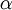$\alpha$ th point mass has mass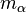$m_\alpha$ , and is in position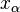$x_\alpha$. In addition to the mutual gravitational force, there exists an external $ext$ ext, due to other systems, ensembles, aggregates, and conglomerations etc., which also influence the total force F acting on the point mass . In this case, the ext is not a constant universal Gravitational field but it is the total vectorial sum of fields at x due to all the external to its system bodies and with that configuration at that moment of time, external to its system of N point masses.Total Mass of system =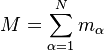$M = \sum_{\alpha=1}^N m_\alpha$ (1)

As it is very difficult for me to type all the equations here , I will just jump to the final equation, Any person wanted to see all the equations can get a copy of full set by email from me.

Total AGGREGATE Equations :( Aggregate consists of many Ensembles and systems)

Assuming these forces are conservative, we can find the resultant force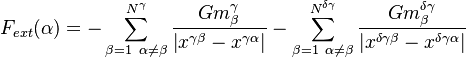$F_{ext}(\alpha) = -\sum_{\beta=1 \ \alpha\ne\beta}^{N^\gamma}\frac{G{m_ \beta^\gamma}}{\left \vert x^{\gamma \beta}-x^{\gamma \alpha} \right \vert} -\sum_{\beta=1 \ \alpha\ne\beta}^{N^ {\delta\gamma}}\frac{G{m_ \beta^ {\delta\gamma}}}{\left \vert x^{\delta \gamma \beta}-x^{\delta \gamma \alpha} \right \vert}$ (2)